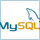# How to use ALTER COMMAND in SQL - database tutorial

In this SQL tutorial  we will see how to use ALTER command command in table on database. ALTER command is mainly used to add, modify and drop columns, indexes and constraints on table in relational database e.g. MySQL, Oracle, Sybase and SQL Server.  Though ALTER is not part of classical CRUD operation but it’s one of the important DDL command. One of the most frequent use of ALTER command in SQL is adding and removing indexes to improve performance of SQL SELECT queries. In this SQL and database tutorial we will see different ways of using ALTER command. By using ALTER command we can add, edit or modify table, views and databases. We can add new column on the table, we can change value of column or we can rename column also.

## 10 ALTER command Example in SQLLet’s see some of the most frequently used example of ALTER command in SQL. In this section we will see How to use ALTER command to add, modify and drop existing columns in table.

Example 1 : How to add column in existing table using ALTER clause in SQL:
Suppose we have one table called student with field roll_number, stu_name, class, subject and we want to add one more column called marks-obtained, how will do using ALTER Command.

ALTER TABLE Student ADD marks_obtained Number (3);

When this field is added to the table it will contain NULL Value by default.

Example 2 :  How to modify existing column using ALTER command in SQL:
Suppose now we want to modify above added field mark_obtained from number to decimal data type we can use modify column along with alter command.

ALTER TABLE Student ALTER COLUMN marks_obtained DECIMAL (5, 2);

Example 3 :  How to drop existing column from table using ALTER command in SQL:
Now if we want to drop some existing column from the table we use drop along with ALTER command

ALTER TABLE Student DROP column marks_obtained;

Example 4. How to drop Primary Key Constraint using ALTER command in database :
Suppose there is a PRIMARY KEY CONSTRAINT named 'pk_roll_num' for the column roll_number of the Student table and we want to drop this constraint

ALTER TABLE Student DROP CONSTRAINT 'pk_roll_num’;

Example 5. How to add primary key constraints using ALTER command in SQL:
Now if we want to add primary key constraint in table Student named 'pk_roll_num' for the column roll_number' . We do like this

ALTER TABLE Student ADD Constraint 'pk_roll_num PRIMARY KEY(roll_number)

Example 6. How to drop foreign key Constraint using Alter command:

Suppose there is a foreign key constraint named 'fk_roll_num' for the column roll_number' of the 'Marks' table and we want to drop this constraint

ALTER TABLE Marks DROP CONSTRAINT 'fk_roll_num’;

Example 7 : How to add foreign key Constraints using alter command
Now if we want to add foreign key constraint in table Marks named 'fk_roll_num' for the column roll_number' .we do like this

ALTER TABLE Marks ADD Constraint 'fk_roll_num FOREIGN KEY (roll_number) REFERENCES Students (roll_number);

Example 8 : How to add unique key Constraints using ALTER command
Now if we want to add Unique key constraint in table Student named ‘unique_roll_no ' for the column roll_number' .we do like this

ALTER TABLE Student ADD CONSTRAINT unique_roll_no UNIQUE (roll_Number);

Example 9 : How to drop unique Key Constraint using ALTER command
Now if we want to drop unique key constraint in table Student named ‘unique_roll_no ' for the column roll_number' .we do like this

ALTER TABLE Student DROP CONSTRAINT unique_roll_no);

Example 10 : How to add check Constraint using ALTER command in SQL
Basic syntax for adding check constraint in table is like this

ALTER TABLE table_name ADD CONSTRAINT MyUniqueConstraint CHECK (CONDITION);

This is all about ALTER command examples in SQL. We have seen 10 different ways of using ALTER command in SQL. ALTER is one of the most important while working in any database e.g. MySQL, Oracle or SQL Server, especially from command line. Since we often need to add, modify and drop columns, indexes and constraints in table.

Further Learning
Introduction to SQL
The Complete SQL Bootcamp
SQL for Newbs: Data Analysis for Beginners

Other SQL and database tutorials and Interview questions

P.S. - If you are looking for online training/course to learn SQL from scratch, I suggest you joining Introduction to SQL by Jon Flanders. It's one of the best sourse to learn SQL fundamentals e.g. join, subquery, aggregate functions, window functions, gropuing data, advanced filtering and SQL query optimization.